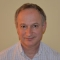Small matters matter. Imagine saving (or spending wisely) just 1 second of your time every hour. One measly second! During your lifespan you would save or spend wisely (1 sec-an-hour * 24 hours-a-day * 365 days-a-year * 100 years) / (3600 seconds-an-hour * 24 hours-a-day) = 10 days, a whole two week vacation!

While truncation vs rounding may seem to be insignificant in a given instance, the cumulative effect of either could be truly enormous, whether it’s truncation vs rounding of decimal numbers or of the SAS time values presented below.

From my prior post Truncating decimal numbers in SAS without rounding, we know that SAS formats such as w.d, DOLLARw.d, and COMMAw.d do not truncate decimal numbers, but rather round them.

However, SAS time value formats are somewhat different. Let’s take a look.

Suppose we have a SAS time value of '09:35:57't. As a reminder, a SAS time value is a value representing the number of seconds since midnight of the current day. SAS time values are between 0 and 86400.

## TIMEw.d Format

Let’s apply the TIMEw.d format to our time value and see what it does.

If you run the following SAS code:

```data _null_; t = '09:35:57't; put t= time5.; put t= time2.; run;```

you will get in the SAS log:

```t=9:35 t=9```

which means that this format does truncate both seconds and minutes. Conversely, if rounding were taking place we would have gotten:

```t=9:36 t=10```

## HHMMw.d Format

Let’s run the same SAS code with HHMMw.d format:

```data _null_; t = '09:35:57't; put t= hhmm5.; put t= hhmm2.; run;```

SAS log will show:

```t=9:36 t=9```

What does that mean? It means that HHMMw.d format rounds seconds (in case of truncating I would expect to get t=9:35), but truncates minutes (in case of rounding I would expect to get t=10, as 35 minutes are closer to 10 than to 9). A bit inconsistent, at least for our purposes.

## Truncating SAS time values

This little research above shows that out of the two formats, TIMEw.d and HHMMw.d, it is perfectly fine to use the TIMEw.d format for the purpose of SAS time value truncation, for both minutes and seconds.

Regardless of the format used, you can also truncate your time value computationally, before applying a format, by subtracting from that value a remainder of division of that value by 60 (for seconds truncation) or by 3600 (for minutes truncation). For example, the following code:

```data _null_; t = '09:35:57't; t_m = t - mod(t,60); t_h = t - mod(t,3600); put t= hhmm5.; put t_m= hhmm5.; put t_h= hhmm5.; run;```

produces the following SAS log:

```t=9:36 t_m=9:35 t_h=9:00```

## Rounding SAS time values

Now that we’ve learned both the computational method and the TIMEw.d format method of truncation, how do we go about rounding? As long as the format behavior is consistent we can use its truncating functionality to convert it into the rounding functionality. In order to do that we just can increase the original time value by 30 (60/2 seconds) for seconds rounding, and by 1800 (3600/2 seconds) for minutes rounding. Truncation of that new value is equivalent to rounding of the original value.

Let’s run the following SAS code:

```data _null_; t = '09:35:57't; t_m = t + 60/2; t_h = t + 3600/2; put t_m= time5.; put t_h= time2.; run;```

SAS log will show:

```t_m=9:36 t_h=10```

which means that our original time value '09:35:57't was rounded in both cases – seconds rounding and minutes rounding.

Now you know how to truncate and how to round SAS time values. And don’t forget about your lifetime 2-week vacation opportunity by saving a second every hour; or make it 2 seconds per hour and enjoy the full month off.

P.S. Our reader Erik Tilanus suggested quite effective use of the ROUND function: for rounding minutes to use round(time, 60) and for rounding hours to use round(time, 3600) as in the following example:

```data _null_; t = '09:35:57't; t_m = round(t,60); t_h = round(t,3600); put t_m= time5.; put t_h= time2.; run;```

Again, SAS log will show rounded values:

```t_m=9:36 t_h=10```

There are some other interesting uses of ROUND function showing how rounding can be done to fractional time intervals.

ShareLeonid Batkhan is a long-time SAS consultant and blogger. Currently, he is a Lead Applications Developer at F.N.B. Corporation. He holds a Ph.D. in Computer Science and Automatic Control Systems and has been a SAS user for more than 25 years. From 1995 to 2021 he worked as a Data Management and Business Intelligence consultant at SAS Institute. During his career, Leonid has successfully implemented dozens of SAS applications and projects in various industries. All posts by Leonid Batkhan >>>

1.Miguel Angel on

Hello Leonid,

Thanks a lot, concise and very clear post regarding date truncation.

Regards,

•Thank you, Miguel, I really appreciate your feedback.

2.Truncating or rounding? Once upon a time records where lost translated to an exterenal DBMS. It was caused by rounding datetime values hitting some of them the representation 24:00:00:00 being invalid at the other end. Surprises of big data as of the size every not so expected situation still will happen.

•Jaap, thanks for your comment. However, in this blog post I only cover output representation of the time values - truncated vs. rounded.

3.Hello

Coding in SAS, how to get better at it?

Thanks
Maria

•Maria,
In short, the answer is 1) learn; 2) practice; 3) apply.

This site uses Akismet to reduce spam. Learn how your comment data is processed.